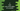# C program to find the sum of digits of a number## C program to find the sum of digits of a number:

In this post, we will learn how to find the sum of the digits of a number in C. The program will take one number as an input from the user and it will print the sum of the digits of that number.

We will learn different ways to find the sum.

### Method 1: By using a while loop:

A for loop or a while loop or a do-while loop can be used to iterate over the digits of a number. We can initialize another variable as 0 to hold the sum of the digits and on each iteration, we can add the digit to this variable to find the final sum.

The below program is using a while loop to find the sum of all digits:

``````#include <stdio.h>

int getSumDigits(int no)
{
int sum = 0;

while (no)
{
sum += no % 10;
no /= 10;
}

return sum;
}

int main()
{
int n;
printf("Enter the number: ");
scanf("%d", &n);

printf("Sum of all digits of %d is %d\n", n, getSumDigits(n));
return 0;
}``````

Here,

• It is asking the user to enter a number. This number is stored in the variable n.
• getSumDigits method is used to get the sum of digits of a number. It takes an integer value as its parameter and returns the sum of digits of that number.

• The sum variable is initialized as 0 to hold the sum of all digits.
• The while loop will run until the value of no is greater than 0. Inside the loop, we are finding the last digit of the number. This digit is added to the sum variable and removes the last digit of the number by dividing the number by 10.
• It returns the final sum.

If you run this program, it will give output as like below:

``````Enter the number: 1243
Sum of all digits of 1243 is 10

Enter the number: 0
Sum of all digits of 0 is 0

Enter the number: 99
Sum of all digits of 99 is 18``````

### Method 2: By using a for loop:

We can also use a for loop to find the sum of digits of a number.

``````#include <stdio.h>

int getSumDigits(int no)
{
int sum = 0;

for (; no; no /= 10)
sum += no % 10;

return sum;
}

int main()
{
int n;
printf("Enter the number: ");
scanf("%d", &n);

printf("Sum of all digits of %d is %d\n", n, getSumDigits(n));
return 0;
}``````

Here,

• getSumDigits method is used to find the sum of all digits of the number.
• This method uses a for loop to find the sum. The loop will run until the value of no is greater than 0 and at the end of each step, it divides the number by 10.

If you run this program, it will give similar output.

### Method 3: By using a recursive method:

This problem can be solved by using a recursive method as well. A recursive method calls itself again and again to find the result. We will write a method that will take the number and another value to store the sum as the parameters. It will call itself recursively to find the sum and return it.

``````#include <stdio.h>

int getSumDigits(int n, int sum)
{
if (n == 0)
return sum;

sum += n % 10;
return getSumDigits(n / 10, sum);
}

int main()
{
int n, sum;

printf("Enter the number: ");
scanf("%d", &n);

sum = getSumDigits(n, 0);

printf("Sum of digits of %d is %d\n", n, sum);

printf("\n");
}``````

In this example,

• getSumDigits method is a recursive method. This method is used to find the sum of digits of a number. It takes a number and another value as the sum of digits as its parameters. The sum of digits is passed as 0 for the first call.

• If the number is 0, it returns the sum calculated.
• Else, It adds the last digit of the number to the sum variable.
• This method is called recursively with n/10 and the same sum variable is passed.
• The return value of this method is the final sum. This is stored in the variable sum and it is printed to the user.

If you run this program, it will print output as like below:

``````Enter the number: 9987
Sum of digits of 9987 is 33

Enter the number: 1234
Sum of digits of 1234 is 10

Enter the number: 123887
Sum of digits of 123887 is 29``````